# @SORT (Sort Series/Groups)

This macro can be used to sort series or groups in a chart.

SYNTAX:

 `@SORT nSort`

PARAMETERS:

nSort; 0...9 selects one of the following sorting options:
0 = Sort Series Labels in Alphabetical order (a...z)
1 = Sort Series Labels in Reverse Alphabetical order (z...a)
2 = Sort Groups Labels in Alphabetical order (a...z)
3 = Sort Groups Labels in Reverse Alphabetical order (z...a)
4 = Sort Series Totals in ascending numeric value (i.e. total all values in each series. Then 'rank' the series from smallest total to largest).
5 = Sort Series Totals in descending numeric value
6 = Sort Groups Totals in ascending numeric value
7 = Sort Groups Totals in descending numeric value
8 = First Series Key to Groups Ascending
9 = First Series Key to Groups Descending

EXAMPLE:

 ``` @DATATEXT 1@RDT 3@SORT 0 ```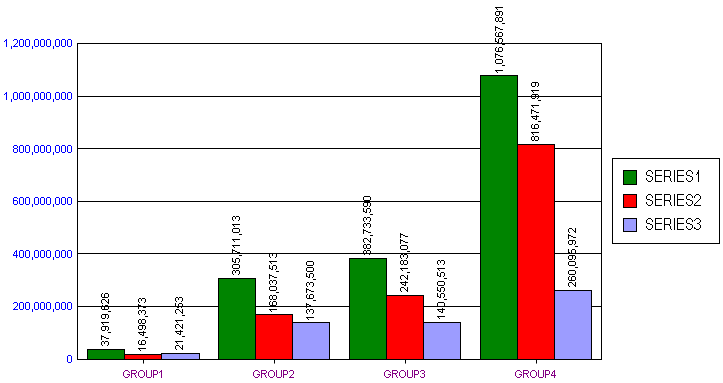```@SORT 1 ```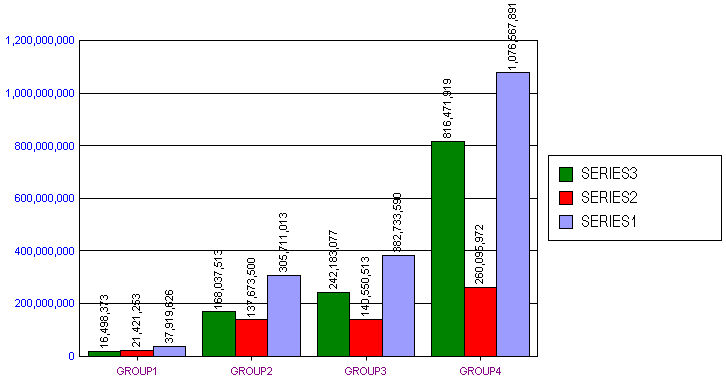```@SORT 2 ```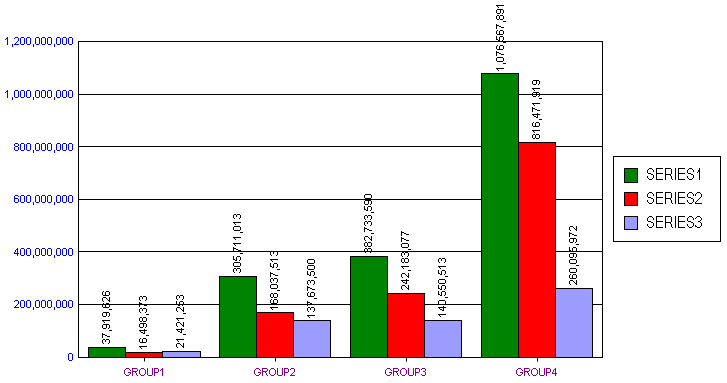```@SORT 3 ```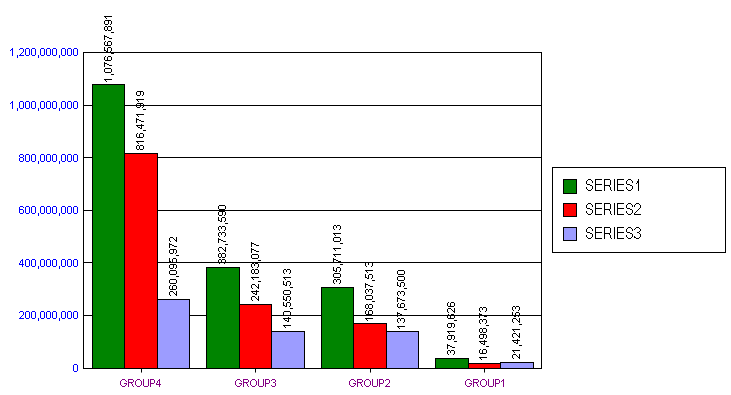```@SORT 4 ```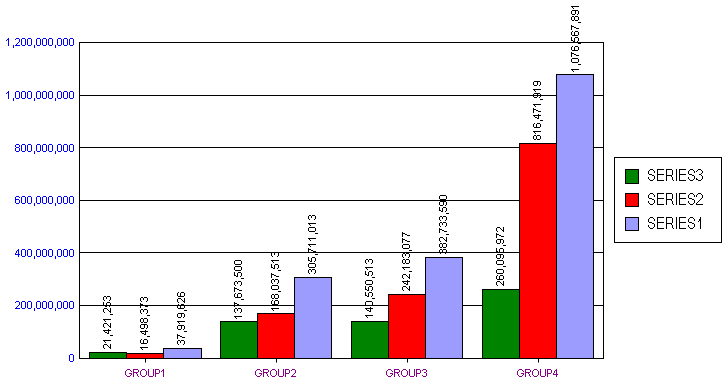```@SORT 5 ```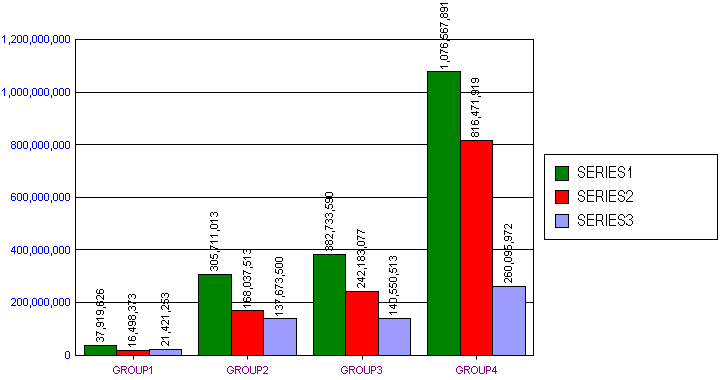```@SORT 6 ```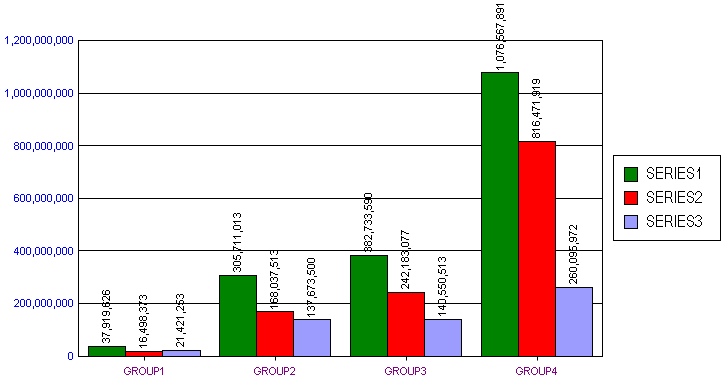```@SORT 7 ```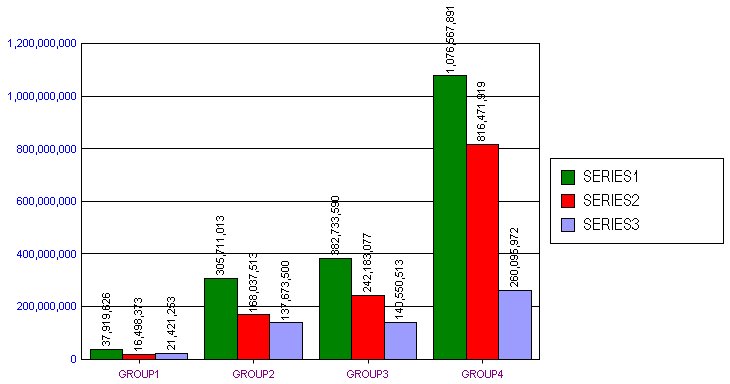```@SORT 8 ```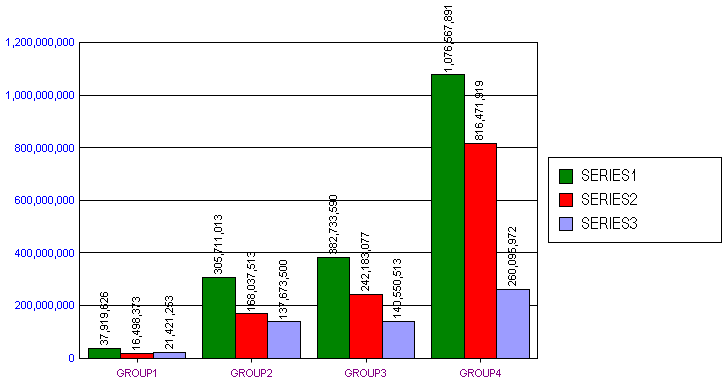```@SORT 9 ```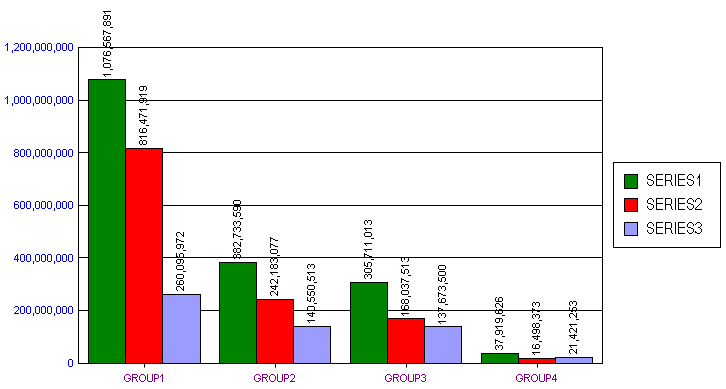PERSISTENT:

NO

 ``` ```

Next MacroPrevious MacroReturn to Alpha List

Next Macro in this GroupPrevious Macro in this GroupReturn to Group List